# TDI Matrices

1. Given an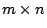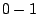matrix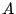and an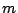-dimensional vector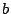, decide whether the system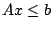is totally dual integral (TDI), that is, is there an integer dual solution for every objective function for which the dual optimum exists.

This is thespecial case of the well-known problem of TDI system recognition. Let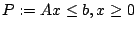. Let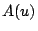be the matrix whose rows are the normal vectors of facets of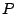containing u, each row is integer and the gcd of its entries is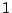.

There are several known relations between TDI and unimodular systems. As Serkan Hosten pointed out, `nondegenerate' TDI matrices are exactly those in whichis an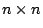matrix having determinantfor every vertex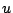.

The following problem involves unimodularity in perfectness test:

INPUT:0-1 matrix A and an-dimensional positive vector,

QUESTION: Is the matrix A(u) for every vertex u of P a square matrix of determinant 1 ?

2. Can this problem be solved in polynomial time ?

For reducing the test for the perfectness of matrixto this problem define b with a 'lexicographic perturbation' from the all 1 objective function.

Contributed by András Sebo

Back to the main index for Perfect Graphs.